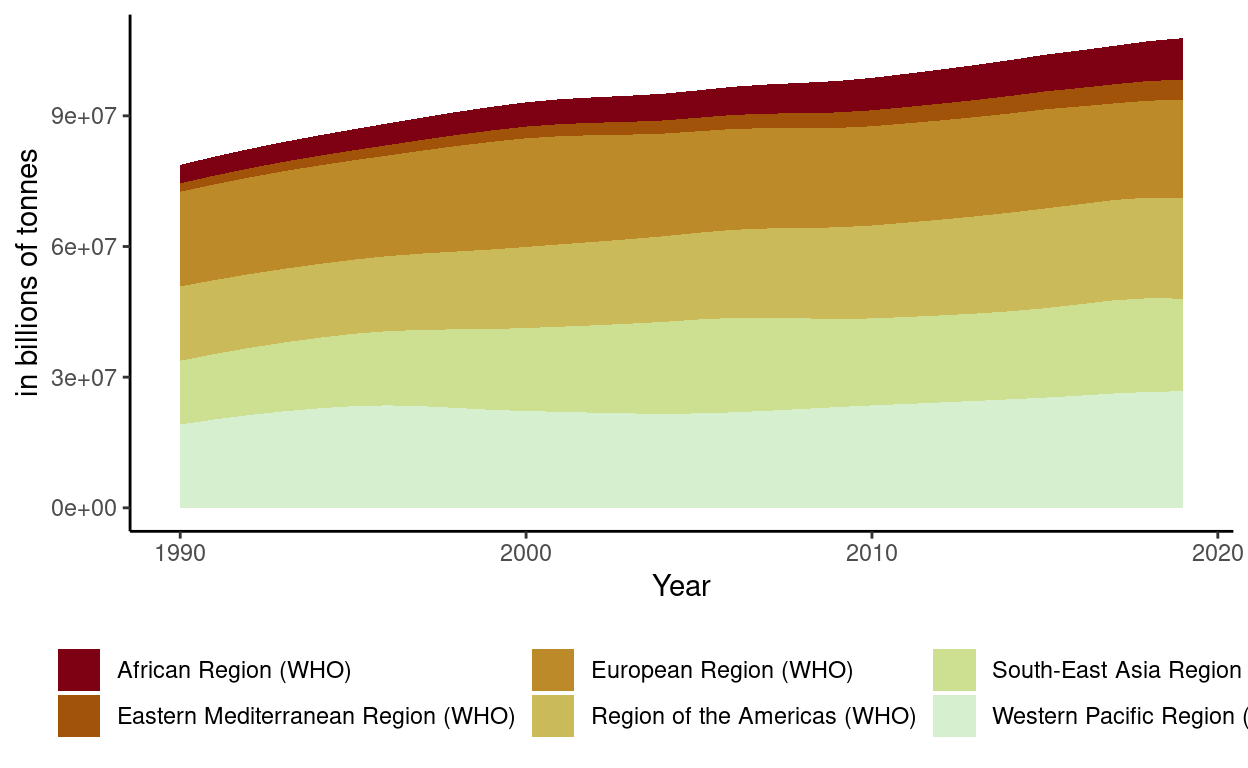# Project Part 2

Interactive and static plots of alcohol use disorder from 1990 to 2019

1. Packages I will use to read in and plot the data
``````library(tidyverse)
library(echarts4r)
``````
1. Read the data in from part 1
``````regional_disorders<-
``````

## Interactive Graph

• Group_by region so there will be a “river” for each region

• Use mutate to round NumberofPeople only 2 digits will be displayed when you hover over it.

• Use mutate to change Year so it will be displayed as end of year instead of beginning of year

• Use e_charts to create an e_charts object with Year on the x axis

• Use e_river to build “rivers” that contain NumberofPeople by region. The depth of each river represents the amount of emissions for each region.

• Use e_tooltip to add a tooltip that will display based on the axis values

• Use e_title to add a title, subtitle, and link to subtitle

• Use e_theme to change the theme to roma

``````regional_disorders    %>%
group_by(Region)  %>%
mutate(
Year = paste(Year, "12", "31", sep="-"))  %>%
e_charts(x = Year)   %>%
e_river(serie = NumberofPeople, legend=FALSE)  %>%
e_tooltip(trigger = "axis")  %>%
e_title(text = "Annual Number with Alcohol Disorders",
subtext = "(in billions of tonnes). Source: Our World in Data",
left = "center")  %>%
e_theme("roma")
``````

## Stactic Graph

• Use ggplot to create a new ggplot object. Use aes to indicate that Year will be mapped to the x axis; number with alcohol disorders will be mapped to the y axis; Region will be the fill variable

• geom_area will display NumberofPeople

• scale_fill_discrete_divergingx is a function in the colorspace package. It sets the color palette to roma and selects a maximum of 12 colors for the different regions

• theme_classic sets the theme

• theme(legend.position = “bottom”) puts the legend at the bottom of the plot

• labs sets the y axis label, fill = NULL indicates that the fill variable will not have the labelled Region

``````regional_disorders   %>%
ggplot(aes(x = Year, y = NumberofPeople,
fill = Region)) +
geom_area() +
colorspace::scale_fill_discrete_divergingx(palette = "roma", nmax =11) +
theme_classic() +
theme(legend.position = "bottom") +
labs( y = "in billions of tonnes",
fill = NULL)
``````These plots show that the Number of People with Alcohol Disorders has not had much significant change through out the years. There has been minimal increases and decreases through 1990 to 2019.

``````ggsave(filename = here::here("_posts/2022-05-17-project-part-2/preview.png"))
``````